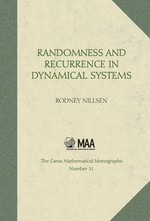# Randomness and Recurrence in Dynamical Systems: A Real Analysis Approach

Rodney Nillsen
Volume: 31
Edition: 1
Pages: 376
https://www.jstor.org/stable/10.4169/j.ctt5hh81m

1. Front Matter
(pp. i-viii)
(pp. ix-xii)
3. Foreword
(pp. xiii-xiv)
Kenneth A. Ross

Mathematics is an ancient discipline, and it persists, and will continue to persist, in a changing world. But as a discipline it is also facing many challenges. At one time, mathematics was a natural choice of university and college study for many serious students. Now there is a vast range of studies and disciplines whose more immediate relationship to society, and the perceived rewards that accompany that immediacy, make mathematics a less natural choice. At the same time, mathematical techniques are permeating more and more disciplines and becoming more essential to those disciplines. This often occurs at the expense of...

4. Preface
(pp. xv-xviii)
Rodney Nillsen
5. Chapter 1 Background Ideas and Knowledge
(pp. 1-23)

LetAandBbe sets and letfbe a function with domainAand codomainB. That is, for each$x\in A$there corresponds$f(x)\in B$. We may indicate this situation symbolically by writing$f:A\to B$. In the case when the domain and codomain are equal to a setSsay, and$f:S\to S$, we say thatfis atransformation on S. In such a case, we call the pair (S, f) adynamical system. Thus, a dynamical system consists of a set together with a transformation on the set. Given a dynamical system (S,f),Smay...

6. Chapter 2 Irrational Numbers and Dynamical Systems
(pp. 25-85)

The discovery of irrational numbers in ancient Greece is attributed to the School of Pythagoras¹ in 500–400 bce. The discovery seems to have caused a type of crisis in Greek science and mathematics, which had until then considered that measurement must consist in counting natural units and considering their ratios—that is, it was considered that measurement is adiscreteprocess, or a process ofcounting. This was the view of Pythagoras, who regarded nature as an expression of whole numbers [5, pp. 154–155]. Sir Karl Popper regarded the discovery of irrational numbers as leading to major philosophical...

7. Chapter 3 Probability and Randomness
(pp. 87-233)

A main aim in this chapter is to pursue a connection between elementary real analysis and probability theory. In probability, we imagine an experiment or a procedure whose set of all possible outcomes is denoted byS. The setSis usually called thesample space. Thus, a pointxinSrepresents a possible outcome of the experiment. Now, consider a subsetAofS. If the experiment is carried out and produces an outcomex, we can observe whether$x\in A$or$x\notin A$. For this reason,Ais often called anevent. Then, if the experiment is carried...

8. Chapter 4 Recurrence
(pp. 235-287)

Imagine an experiment where an unbiased coin is tossed at random over and over again. Then, recurrence in this process is illustrated by the fact that, sooner or later, we expect to get the outcome “heads”. What is more, if we continue tossing, sooner or later we expect to get the result “heads” again—the outcome “heads” will then haverecurred.

More generally, imagine a process developing over time. One state of the process may be observed and then, at a later time, the same state, or one close to it, may be observed again. We might say that the...

9. Chapter 5 Averaging in Time and Space
(pp. 289-350)

This chapter of the book is different from the preceding Chapters 2, 3 and 4. Its role is primarily expository, and introduces more advanced ideas, concepts, results and topics that proceed naturally from the preceding chapters. In particular, there is an exposition of parts of measure theory, and this is used to revisit some earlier topics and ideas, and to view them through the powerful lens measure theory provides. Some proofs are included, but most are omitted. An aim is also, in part, to present some motivations and underlying ideas in measure theory and ergodic theory that may not be...

10. Index of Subjects
(pp. 351-354)
11. Index of Symbols
(pp. 355-356)